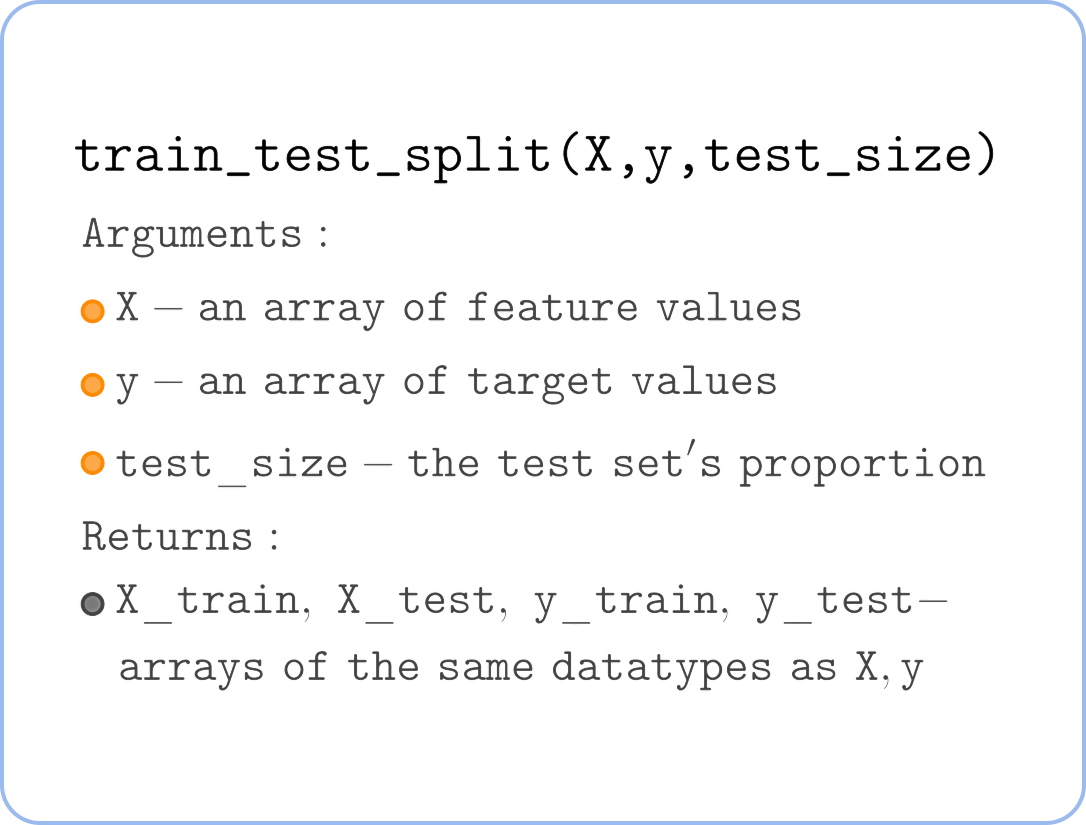Course Content

# Linear Regression with Python

Linear Regression with Python

##Challenge

For this challenge, you will build the same Polynomial Regression of degree 2 as in the previous challenge. However, you will need to split the set into a training set and a test set to calculate RMSE for both those sets. This is required to judge whether the model overfits/underfits or not.
Here is the reminder of the `train_test_split()` function you'll want to use.And also reminder of the `mean_squared_error()` function needed to calculate RMSE:

Now let's move to coding!

1. Assign the DataFrame with a single column `'age'` of `df` to the `X` variable.
2. Preprocess the `X` using the `PolynomialFeatures` class.
3. Split the dataset using the appropriate function from `sklearn`.
4. Build and train a model on the training set.
5. Predict the targets of both training and test set.
6. Calculate the RMSE for both training and test set.
7. Print the summary table.

When you complete the task, you will notice that the test RMSE is even lower than the training RMSE. Usually, models do not show better results on unseen instances. Here, the difference is tiny and caused by chance. Our dataset is relatively small, and while splitting, the test set received a bit better(easier to predict) data points.

Everything was clear?

Section 4. Chapter 4